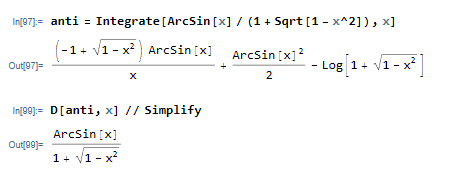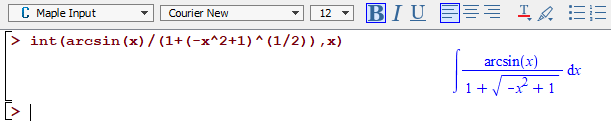## Can one obtain infinity as a solution for a variab...

sometimes I get a solution to an ODE, where when I plugin the initial conditions to solve for the constant of integration, the solution by inspection can be seen to be infinity.

The constant of integration is allowed to be infinity.

But now I get no solution from solve and so my program rejects this solution and says the ode can't be solved because it can't find value for the constant of integration using this initial conditions.

I am not sure how to handle this, since Maple solve will not return an infinity as possible solution for the constant of integration. I tried different assumptions.

This has to work for general, and not for this specific example I will show now.

Here is an ODE with IC

```ode:=diff(y(x),x)=y(x)^3*sin(x);
ic:=y(0)=0;
sol:=[dsolve(ode)];```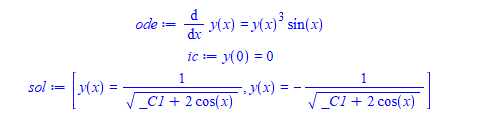The general solution is easy to find since this is just separable. The problem is when I plugin the initial conditions. (again, this is done in a program, without looking at the screen). let consider the first solution above for now.

`eq:=simplify(subs( [y(x)=0,x=0],sol));`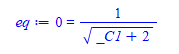And here is the problem, when next I try to solve for the constant, Maple says no solution.

`solve(eq,_C1,allsolutions=true) assuming _C1::real`

No solution returned.

If Maple could return _C1=infinity, then I would plugin _C1=infinity and obtain the correct particular solution which is

`simplify(subs(_C1=infinity,sol)) assuming real`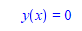Which is what the smart dsolve command does

```ode:=diff(y(x),x)=y(x)^3*sin(x);
ic:=y(0)=0;
sol:=dsolve([ode,ic])```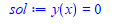I do not know how Maple found the above and what algorithm it used.

But my not too smart dsolve program gets stuck on such problems, because it can't solve for the IC.

Is there another method or option or assumptions to use, to solve such an equation and request infinity be returned as a solution?  I tried isolate(eq,_C1) but that did not work.

One possible option to try as a last resource is to do this

`limit(eq,_C1=infinity)`

And this gives 0=0

which means _C1=infinity is valid solution.  And I could do this as final attempt before giving up. Is this what Maple did? But how does one know to do this in general? Unless one tries and find out? i.e I could try limit(eq,_C1=infinity) and limit(eq,_C1=-infinity) and see if any one of these gives true (after doing  evalb or is  on the output)

Any suggestions or a better/more robust method to use for such cases? I need to use the method for any equation where infinity can be a solution for the constant of integration. It will always be one equation and one variable, and can assume all are real for now if it makes it easier.

## why dsolve do not show this solution?...

I was wondering why dsolve do not show this one solution I obtained different way, for this ode.

Maple gives 6 solutions to the ODE. But 5 of them are signular (have no constant of integration in them). I am looking at the last solution it gives, the one with constant of integration.

But when I solve this ODE, after simplifying it, I get different general solution than the last one Maple shows. I thought they might be equivalent, but I do not see how they could be.

So my question is why Maple did not show the simpler solution also?  Here is the code

`ode_orginal:=1/3*(-2*x^(5/2)+3*y(x)^(5/3))/x^(3/2)*diff(y(x),x)/y(x)^(5/3)+1/2*(2*x^(5/2)-3*y(x)^(5/3))/x^(5/2)/y(x)^(2/3) = 0;`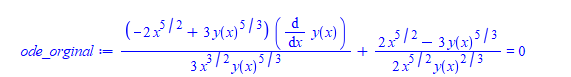This looks complicated and non-linear, but when I solve for y'(x) it gives much simpler and linear ODE (Have to convert the ODE to D before solving for diff(y(x),x), since Maple can complain otherwise).

```ode:=convert(ode_orginal,D);
ode:=diff(y(x),x)=solve(ode,D(y)(x),allsolutions=true);
```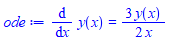And solving the above gives the much simpler solution

```sol:=dsolve(ode,y(x));
odetest(sol,ode);```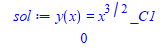Now when using dsolve on the original complicated lookin ODE it gives

```maple_sol:=[dsolve(ode_orginal)];
```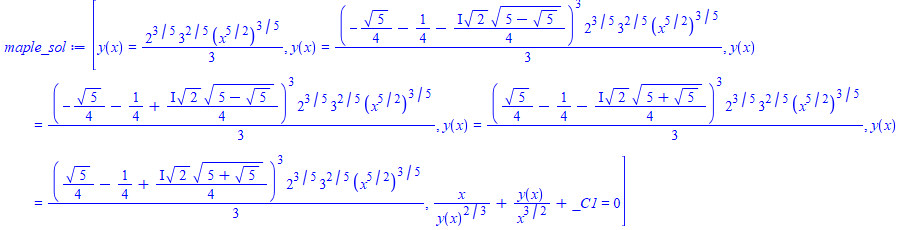if there is any hope that one of the above 6 solutions will be the same as the simpler solution, it has to be the last one, with the _C1 in it, since all the others do not have _C1 (singular solutions).

But solving for y(x) from the last one does not give the simpler solution.

So it is a new general solution for the original ode and it is correct, since odetest gives zero.

I think in the process of solving for y'(x), some solutions got lost. Even though I asked for allsolutions there?.

But my main question is: Should dsolve have also have given the simpler solution? Since that one also satisfies the original nonlinear complicated ode

```odetest(sol,ode_orginal);
```

gives 0.

Maple 2021.1

## why odetest does not verify this solution against ...

I solved this ode with IC, and obtained a solution.  Maple odetest says my solution satisfies the ODE but not the initial conditions. But I do not see why. When I plugin manually the initial conditions into the solution, I get true.

So I am not sure what is going on.

```restart;
ode:=x*diff(y(x),x)*y(x) = (x+1)*(y(x)+1);
ic:=y(1)=1;
mysol:=y(x)-ln(y(x)+1)=-ln(2)+x+ln(x);
my_sol_at_IC:=subs([y(x)=1,x=1],mysol);

#now check manually
evalb(my_sol_at_IC);
is(my_sol_at_IC);
```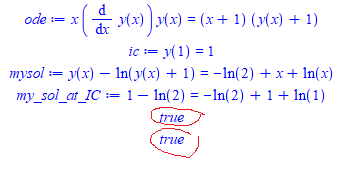Using odetest gives

```odetest(mysol,ode);
odetest(mysol,[ode,ic]);
```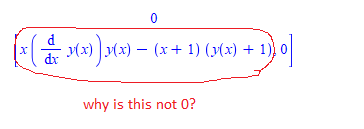What Am I doing wrong?

Maple 2021.1

Edit June 12, 2021

This is another example where odetest gives different result depending on how the solution is written !

```restart;
ode:=diff(y(x),x)=2/3*(y(x)-1)^(1/2):
mysol_1:=y(x) = 1/9*x^2 - 2/9*x + 10/9:
mysol_2:=y(x) - (1/9*x^2 - 2/9*x + 10/9) = 0 :
odetest(mysol_1,ode);
odetest(mysol_2,ode);
```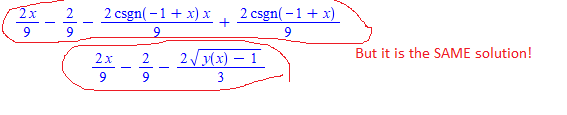## strange result using evalb() in debugger vs. outsi...

I can't figure what is going on here. I have an expression, where when I run the program, I see inside the debugger that the result of evalb(simplify(tmp_1)) where tmp_1 is the expression, gives false

When I copy the same tmp_1 expression to new worksheet and do evalb(simplify(tmp_1)) it gives true.

I added print statements in the code to print tmp_1 and print result of simplify(tmp_1) and for some reason, it gives false when I run the program, but it gives true when I do the same thing in a work sheet, by copying tmp_1 and trying it there.

Never seen anything like this, as expression is all numbers. Here is the print out when I run the program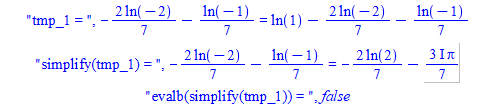Here is the same thing in worksheet

```restart;
tmp_1 :=-(2*ln(-2))/7 - ln(-1)/7 = ln(1) - (2*ln(-2))/7 - ln(-1)/7;
simplify(tmp_1);
evalb(simplify(tmp_1));
```

true

and if you think I made mistake somewhere, here is also screen shot from the debugger window itself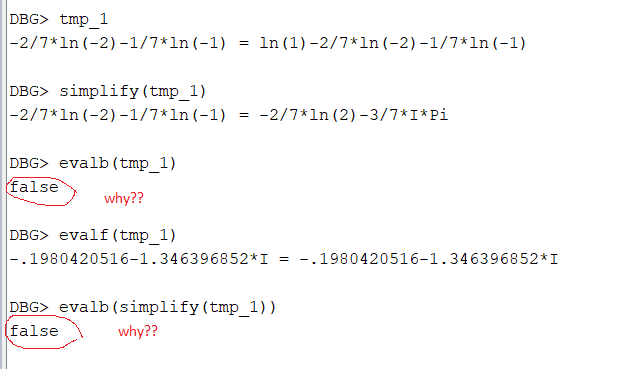again, same thing gives true in separate worksheet, copying same exact expression from above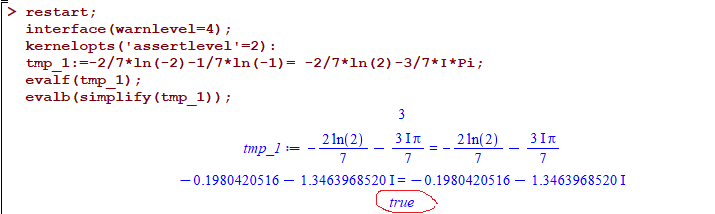There seem to be something loaded when I run my program that causes this difference, but I have no idea now what it is.  This has nothing to do with the debugger loaded btw, I am just using the debugger to show the details. When I run the program, without the debugger, I get false for the above, and I was trying to find why.

Any suggestions what to try and what to check for and what could cause this? Should it not give true when I run the program also? Could it be some Digits setting changed when the program run? I do not change any system defaults of any sort when running the program.

Maple 2020.1

edit june 12, 2021

Here is another manifestation of the same problem I just found. There is something seriously wrong for it to behave this way.

This is an expression called tmp_1 in a local variable in a proc inside a module. There are not even any local symbols in it. When I run the program, all the attempts to show it is true fail. Some give FAIL and some give false when the result should be true

When I simply copy the expression from the debugger window to an open worksheet, I get true

Here is the screen shot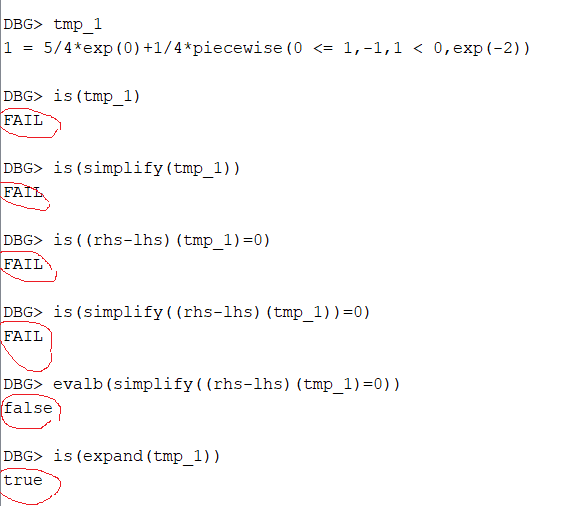Only when I did is(expand(tmp_1)) did it give true

But when I copy the expression to the worksheet, it gives true using all the other attempts:

```restart;
tmp_1:=1 = 5/4*exp(0)+1/4*piecewise(0 <= 1,-1,1 < 0,exp(-2)):
is(tmp_1);
is(simplify(tmp_1));
is((rhs-lhs)(tmp_1)=0);
is(simplify((rhs-lhs)(tmp_1)=0));
evalb(simplify((rhs-lhs)(tmp_1)=0));
is(expand(tmp_1))
```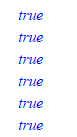I can't make a MWE so far, since these problems only show up with I run my large program. I think Maple internal memory get messed up or something else is loaded that causes these stranges problems.

For now, I added expand() to the things I should try. My current code now looks like

```if is(tmp_1) or is(simplify(tmp_1)) or is((rhs-lhs)(tmp_1)=0)
or is(simplify((rhs-lhs)(tmp_1)=0)) or evalb(simplify((rhs-lhs)(tmp_1)=0))
or is(expand(tmp_1)) then
....
```

Maple 2021.1

## Two remarks about discrete distributions in Maple...

by: Maple 2021

... and two suggestions to the development team

POINT 1
In ?DiscreteValueMap (package Statistics) it's given an example concerning rhe Geometric distribution along with this comment:
"The Geometric distribution is discrete but it necessarily assumes integer values, so (bold font is mine) it also does not have a DiscreteValueMap"

This sentence seems to indicate that "because a distribution is discrete over the set of integers, it cannot have a DiscreteValueMap", some sort of logical implication...

But my feeling is that the Geometric distribution (or any other discrete distribution) does not have a DiscreteValueMap because this attribute has just not been specified when defining the distribution.

```restart:
with(Statistics):

GeomRV := RandomVariable(Geometric(1/2)):
f := unapply(ProbabilityFunction(GeomRV, n), n):

AnotherGeomRV := Distribution(
'ProbabilityFunction'=f,
'Support'=0..infinity,
'DiscreteValueMap'=(n->n),
'Type'=discrete
):
DiscreteValueMap(AnotherGeomRV , n);
```

Thus having the set of natural numbers as support doesn't imply that DiscreteValueMap cannot exist.

Suggestion 1: modify the ?DiscreteValueMap help page so that it no longer suggests that some discrete distributions cannot have a .DiscreteValueMap

______________________________________________________________________________________

POINT 2
I think there exists a true problem with the definition of discrete distributions in Maple: the ProbabilityFunction of a (discrete) random variable) takes non zero values outside their definition set.
For instance

`ProbabilityFunction(GeomRV, Pi);  # something non null`

To ivercome this problem I defined a new Geometric distribution this way (not entirely satisfying):

```restart:
with(Statistics):

GeomRV := RandomVariable(Geometric(1/2)):
f := unapply(ProbabilityFunction(GeomRV, n), n):
g := n -> (1-ceil(n-floor(n)))*f(n)    # (1-ceil(n-floor(n))) = 1 if n in Z, 0 otherwise

AnotherGeomRV := Distribution(
'ProbabilityFunction'=g,
'Support'=0..infinity,
'DiscreteValueMap'=(n->n),  # is wanted
'Type'=discrete
):
ProbabilityFunction(AnotherGeomRV, 2);
1/8
ProbabilityFunction(AnotherGeomRV, Pi);
0
```

PS: None of the statistics based upon the  ProbabilityFunction (Mean, Variance, ... ) is correctly computed with the previous construction. This could be easily overcome by completing this definition, just as its done in Maple, for all the requires statistics, for instance

```AnotherGeomRV := Distribution(
....
'Mean'=1   # or more generally (1-p)/p form Geometric(p)
):```

Suggestion 2: modify the way discrete distributions are defined in Maple in order to avoid ProbabilityFunction to return wrong values.

## how to change subexpression with some specific pat...

I need to change any occurance of   anything*sqrt(anything)  to say Z in any large expression.  (later, I can add the correct replacement once I know how to do it for Z).

I can change   sqrt(anything) with the help of the answers in Substitution-Of-Sub-Expressions-Is  but not  anything*sqrt(anything)

Here is an example to make it clear. Given

`expr:=(-x + sqrt(9*x^2*exp(2*c) + 8)*exp(-c)+99)/(4*x)+ (a*sqrt(z)-99+sin(c*sqrt(r+4)+20))/3+10+1/(c+exp(-x)*sqrt(exp(x)))+sqrt(h)`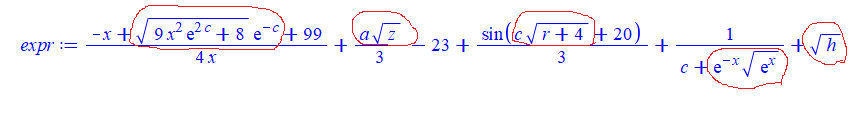Need to all some transformation on  those subexpressions circled above. For now, lets say I wanted to replace them with so it should becomes this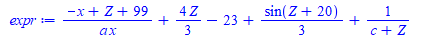I can do this

`subsindets(expr,anything^({1/2,-1/2}),ee->Z)`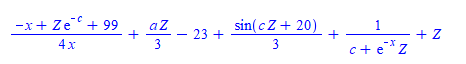But I need to have the term (if any) that multiplies the sqrt as well included.

And that is the problem. Can't figure how to do it. When I try

`subsindets(expr,anything*anything^({1/2,-1/2}),ee->Z)`

Maple says Error, testing against an invalid type Ok. So anything*anything^({1/2,-1/2}) is not a type. What to do then?  Tried also subsindets(expr,t::anything*anything^({1/2,-1/2}),ee->Z) same error

And

```applyrule(t1::anything*sqrt(t0::anything)=Z, expr);
```

gives  which is wrong.

How to make this work in Maple using subsindets? Can one use pattern with subsindets? How to make a type for

anything*sqrt(anything)

Maple 2021.1

## How to suppress axes labels...

I want to plot a 2D graph without labels. The labes=["",""] option does half of the job—it prints the empty string for labels (that's good) but it reserves room for them (that's bad).  In the following code I use a large size labelfont in order to exaggerate the effect:

```restart;
plots:-setoptions(labelfont=[TIMES,64]);  # large labelfont selected on purpose
p1 := plot([[0,0],[1,1]], labels=["", ""]);```Note the large blank space at the bottom reserved for the the non-existent label.

I know one way to eliminate the label altogether:

`p2 := subs(AXESLABELS=NULL, p1);`This does the right job but is there a more orthodox way of doing that?

Afterthought:  It would be good if the labels option to the plot command  accepted none as argument, as in labels=[none, none].

## on output format from solve with exponential...

Why Maple likes to extract exp() outside the sqrt when its argument has minus sign vs. not?  Compare the following

```restart;
eq2 := ln(2*u^2 + u - 1) = -c - 2*ln(x);
sol:=[solve(eq2,u)]:
simplify(sol)
```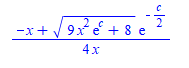and

```eq2 := ln(2*u^2 + u - 1) = c - 2*ln(x);
sol:=[solve(eq2,u)]:
simplify(sol)
```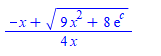I like the above much better than the first one. Mathematica keeps both same form (i.e. keeps the exp() inside):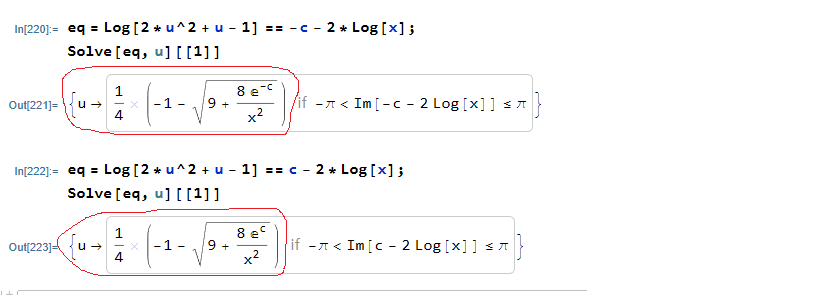Maple's answers are correct ofcourse, I just do not understand the logic why when there is a minus sign on it likes to format it differently as shown.

Is there a way to make not do that?

## Exporting 3D Plot in Vector Graphics Format...

I am trying to export a 3D plot from Maple (2021) in a vector format that can be imported into Adobe Illustrator without too much trouble. The graphic artist says the PDF files are not useful (“at the base of every PDF is an image file, inside layers and layers of clipping paths; not helpful”). “SVG does not appear to produce usable vectors – well, maybe not, but the one you sent me was super difficult to wrangle”. She had hoped that EPS would be useful, but she was unable to open the EPS file I created (not enough memory).

Here is the example that I have been using as I've asked others for help with this question.

```restart;
with(plots);
with(plottools);

T := torus([0, 0, 0], 1/2, 1/2);
C0 := spacecurve([cos(t), sin(t), 0], t = 0 .. 2*Pi, color = red, thickness = 9);
C1 := spacecurve([0.5*cos(t), 0.5*sin(t), 0.5], t = 0 .. 2*Pi, color = red, thickness = 9);
C2 := spacecurve([0.5*(1 + cos(t)), 0, 0.5*sin(t)], t = 0 .. Pi, color = blue, thickness = 9);
C3 := spacecurve([0.5*(-1 + cos(t)), 0, 0.5*sin(t)], t = 0 .. Pi, color = blue, thickness = 9);
Fig9510 := display([T, C0, C1, C2], view = [DEFAULT, DEFAULT, 0 .. 1/2], scaling = constrained, transparency = 0.75);```

Direct links to the worksheet and the PDF, EPS, and SVG files on DropBox are provide below in the hope that something will be useful to somebody. (MaplePrimes links are provided for the MW and PDF files are also provided - but EPS and SVG files are not permitted.)

1. 3DPlotExample.mw: https://www.dropbox.com/s/mx1hg1ntuqihnu3/3DPlotExample.mw?dl=0
2. Fig9510.pdf: https://www.dropbox.com/s/bnzfqrh060gw84g/Fig9510.pdf?dl=0
3. Fig9510.eps: https://www.dropbox.com/s/h9bjyn0uwm8w5ct/Fig9510.eps?dl=0
4. Fig9510.svg: https://www.dropbox.com/s/u2xfntnqvbhu810/Fig9510.svg?dl=0

This question has been asked for more than ten years. The best solution to has been to use the command-line-interface version of Maple.

1. Is the command-line interface still the best/only option for vector-based graphics output?
2. If so, how do i access the command-line version of Maple 2021 on MacOS?

## How to Use Nonexecutable math in documents.?...

The attached worksheet is a stripped down version of some notes I am creating concerning the solution of vector equations in geometric algebra.  For the purposes of explanation it is best to show the math in its conventional typeset form before showing how to solve the equations in maple. The non-excutable math is in the text which is contained in a paragraph inserted before the execution group. In the first instance, the typesetting engine seems content to leave the text alone, but in the second case (which I created in the same way) it insists on parsing the equations and generating errors. I have tried repeatedly to make the second set of equations non-executable (since in maple terms they are nonsense) to no avail.
Can anyone suggest how I can use maple's text features to make the explanatory text inert.

Note that I have included the calls to my geometric algebra module in case they have some relevance to the problem, but they will not execute without the module.

Sample worksheet:  nonexecmatherror.mw

## Error attempting to install Physics Package Update...

When I click on the "install" option for the update to the Physics Package, get the following error message:

Fetching package "Physics Updates" from MapleCloud...
ID: 5137472255164416
Version: 988
URL: https://maple.cloud

An error occurred, the package was not installed:

## Error, (in SumTools:-DefiniteSum:-ClosedForm) nume...

In Maple 2021.1

```restart;
int(cos(3*x)/(-(-1+8*cos(x)^2)^(1/2)+(3*cos(x)^2-sin(x)^2)^(1/2)),x)
```

gives

Error, (in SumTools:-DefiniteSum:-ClosedForm) numeric exception: division by zero

But in Maple 2020.2 it works OK giving an answer. (A very long one)

```3/4*arcsin(2/3*sin(x)*3^(1/2))-3/4*arctan(sin(x)/(-1+4*cos(x)^2)^(1/2))-3/4*arctan(sin(x)/(-1+8*cos(x)^2)^(1/2
))+5/8*arcsin(2/7*sin(x)*14^(1/2))*2^(1/2)-1/2*sin(x)*(-1+4*cos(x)^2)^(1/2)-1/2*sin(x)*(-1+8*cos(x)^2)^(1/2)```

Is this a known issue? I know Maple int has went some changes and improvements in Maple 2021 from the release notes. May be this was caused by some of these changes?

https://www.maplesoft.com/products/maple/new_features/

• Integration has been enhanced with improved algorithms for indefinite integration, and the ability to easily specify which integration method should be used and to compare the results from different methods."

Maple 2021.1 on windows 10

update July 2, 2021

These are additional failed integration in 2021.1 that throw exceptions now. no errors in 2020.2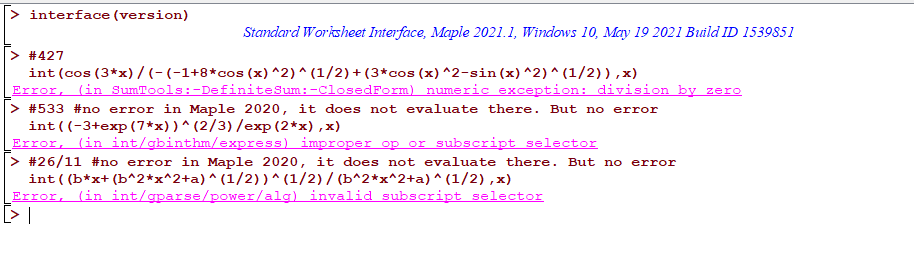```#427
int(cos(3*x)/(-(-1+8*cos(x)^2)^(1/2)+(3*cos(x)^2-sin(x)^2)^(1/2)),x)

#533 #no error in Maple 2020, it does not evaluate there. But no error
int((-3+exp(7*x))^(2/3)/exp(2*x),x)

#26/11 #no error in Maple 2020, it does not evaluate there. But no error
int((b*x+(b^2*x^2+a)^(1/2))^(1/2)/(b^2*x^2+a)^(1/2),x)
```

## why dfieldplot and phaseportrait and DEplot not ac...

dsolve accept system of first order ode's in the form x'=A x, where x' is vector, A is matrix of coefficients and x are the dependent variables. This is convenient since one does not have to convert things to a list.

But  dfieldplot and phaseportrait and DEplot do not accept this form. One must convert things to list first.

Here is an example

```restart;
sys:=Vector([diff(x(t),t),diff(y(t),t)]) = Matrix([[1,2],[0,3]]).Vector([x(t),y(t)]);
dsolve(sys)
```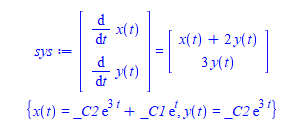But

```DEtools:-dfieldplot(sys,[x(t),y(t)],t=0..4,x=-4..4, y=-4..4);
```

Error, (in DEtools/dfieldplot) system must have same number of dependent variables as DE's.

workaround is to write the system as list

```new_sys:=[diff(x(t), t)=x(t) + 2*y(t),diff(y(t), t)=3*y(t)];
DEtools:-dfieldplot(new_sys,[x(t),y(t)],t=0..4,x=-4..4, y=-4..4);
```

I know one can automate the conversion. But still, it would be better if dfieldplot would accept sys as dsolve did.

Same for

```DEtools:-phaseportrait(sys,[x(t),y(t)],t=0..4,[[x(0)=1,y(0)=0]],x=-4..4, y=-4..4);
#
DEtools:-DEplot(sys,[x(t),y(t)],t=0..4,[[x(0)=1,y(0)=0]],x=-4..4, y=-4..4);
```

They gives same error.

Since dsolve can do it, may be these other functions can also support taking a system of ode's in vector/matrix form? Any reason why not?

Maple 2021.1

## Possible bug in seq(), add(), mul()...

There seems to be an "issue" when using a indexed name (say x) as the loop index in a seq() mul() or add() command - the indexed name is assigned once the command exits!!

This never(?) happens if the loop index is not an indexed name. A bug??

See the attached which illustrates the issue for the add() command, where x is assigned on exit from add(). The same thing happens if add() is replaced with seq() or mul()

 > restart;
 > # # x should not be assigned on exit from add() !! #   add( u(x), x=1..10);   x; # # x__4 is not assigned on exit from add() #   add( u(x__4), x__4=1..10);   x__4; # # j is not assigned on exit from add() #   add( u(j), j=1..10);   j;(1)
 >

## Error, (in Utils:-TransformAndApply) numeric excep...

I do not remember if there was a post on this before or not
Maple 2021.1

```int(arcsin(x)/(1+(-x^2+1)^(1/2)),x)
```

gives

Error, (in Utils:-TransformAndApply) numeric exception: division by zero

`((-1 + Sqrt[1 - x^2]) ArcSin[x])/x + ArcSin[x]^2/2 -  Log[1 + Sqrt[1 - x^2]]`